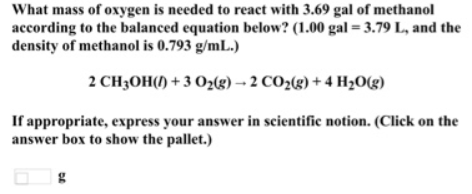# Problem: What mass of oxygen is needed to react with 3.69 gal of methanol according to the balanced equation below? (1.00 gal = 3.79 L, and the density of methanol is 0.793 g/mL.) 2CH3OH() + 3O2(g) → 2CO2(g) + 4H2O(g)If appropriate, express your answer in scientific notion. (Click on the answer box to show the pallet.)

###### FREE Expert Solution
94% (389 ratings)###### Problem Details

What mass of oxygen is needed to react with 3.69 gal of methanol according to the balanced equation below? (1.00 gal = 3.79 L, and the density of methanol is 0.793 g/mL.)

2CH3OH() + 3O2(g) → 2CO2(g) + 4H2O(g)

If appropriate, express your answer in scientific notion. (Click on the answer box to show the pallet.)